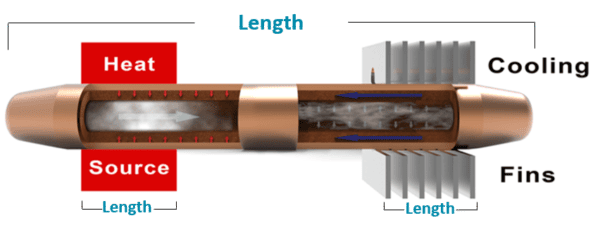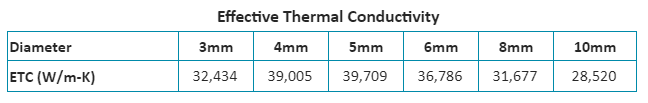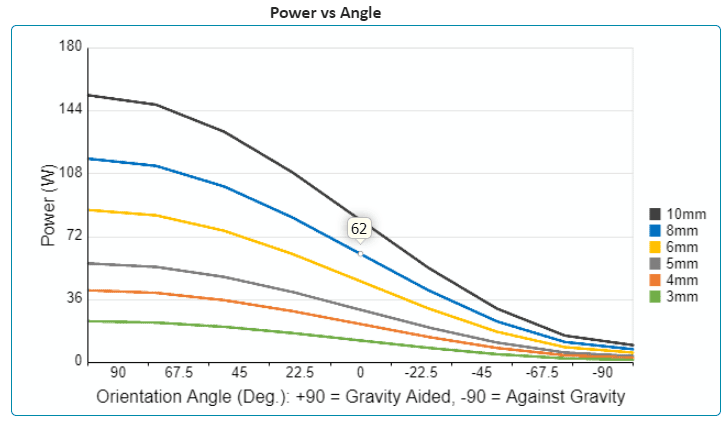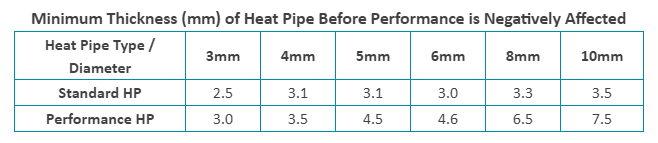This calculator provides three pieces of information based on heat pipe dimensions and operating temperature: the effective thermal conductivity for use in CFD modeling, maximum power handling (Qmax) based on the angle of operation, and possible heat pipe alternatives based on the delta-T at various input powers.  Please note this is for copper/water heat pipes that use a sintered wick structure.

Here’s a link to the online calculator.

The reason for publishing this blog has far more to do with how to read the results of the calculator than it does with the input section. Nonetheless, I’ll still briefly cover the latter.

Heat Pipe Length – the full length of the heat pipe if the evaporator is at one end.Evaporator Length – the evaporator length is the length of the actual heat source.

Condenser length – the distance between the points where the heat pipe enters and exits the condenser.

Heat Pipe Type – Select between ‘Standard’ wick and ‘Performance’ wick. Standard wicks will allow you to flatten the heat pipe more before affecting the maximum power (Qmax). Note that we can alter the wick porosity and thickness to closely match the requirements of your application.

Operating Temperature – This one is tricky because it involves both the liquid and vapor temperatures inside the active heat pipe. In other words, you won’t know it. However, can use a good surrogate by adding 15 deg C to the max ambient temperature at which the device is designed to operate.

THE RESULTS

The first table is for the effective thermal conductivity of heat pipes from 3-10mm. We probably should have put this last as it’s used once you’ve selected the correct diameter. Nonetheless, this figure is used as input into CFD modeling software such as FloTHERM.Next, there is a Power vs Angle of Operation graph (shown) and corresponding table (not shown). Essentially this is giving you the maximum power handling capacity (Qmax) of a particular diameter heat pipe at various angles.  At “+90” degrees the condenser is directly above the evaporator, making it very easy for the condensed vapor (water) to return to the evaporator, hence the high Qmax.There are a couple of design guidelines that will come in handy at this point. First, heat pipe Qmax is additive, provided each heat pipe(s) is over the heat source. In our example, this means that one 8mm heat pipe has a Qmax of 62 degrees Celsius in horizontal operation, while two 8mm pipes have a Qmax of 124 deg. C. Second, build in a safety factor to avoid running the pipe at maximum capacity. Derating the heat pipe Qmax by 20% is a good industry standard – in this case a single pipe would be rated at just under 50 watts. Occasional, short power spikes above this are OK so long as they are still below the rated Qmax.

The Power vs Delta-T graph and table (shown below) needs to be used in conjunction with the above graph. Let’s say we’ve selected the 8mm heat pipe we talked about earlier: in the horizontal position, it will safely carry just under 50 watts (after being derated).  If we put 40 watts into one end, the other end will have a delta-T of 4.3 deg C (lower is better).  Let’s assume we wanted to use two 8mm heat pipes. In that case we could safely double our heat input to 80 watts. However, then using the chart we would still use the 40 watt input figure because each heat pipe would carry 40 watts and the delta-T of each heat pipe would be the same 4.3 degrees C.

The last bit of information given isn’t a calculation, it’s simply some guidance on how much you can flatten heat pipes of different diameters before the Qmax will be negatively affected. The reason the performance heat pipes suffer degradation before standard pipes has to do with the thicker wick structure of the former.